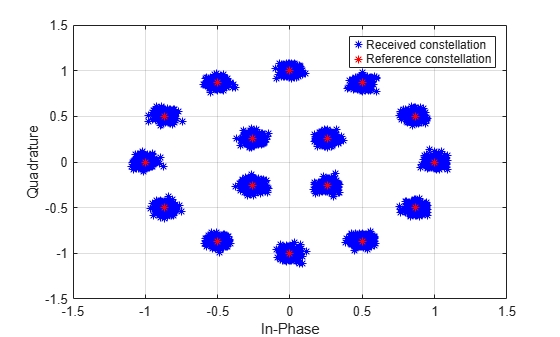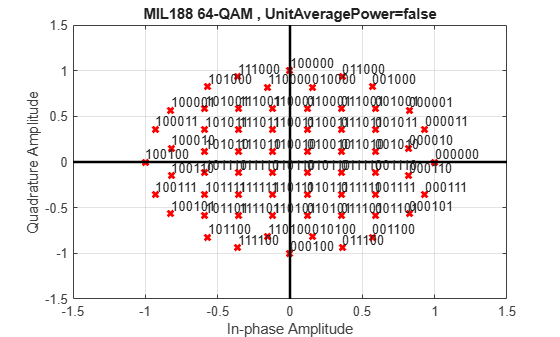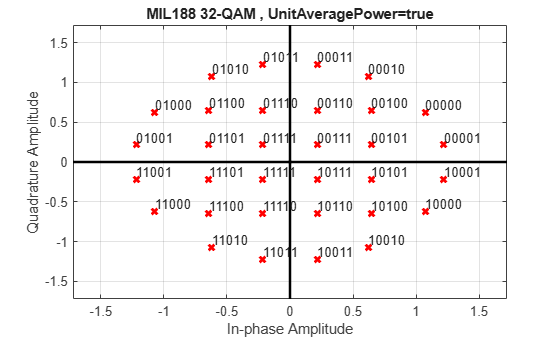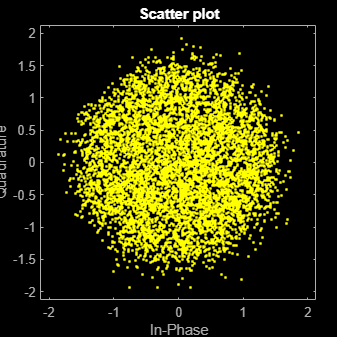# mil188qamdemod

MIL-STD-188-110 B/C standard-specific quadrature amplitude demodulation

## Syntax

``z = mil188qamdemod(y,M)``
``z = mil188qamdemod(y,M,Name,Value)``

## Description

example

````z = mil188qamdemod(y,M)` performs QAM demodulation on an input signal, `y`, that was modulated in accordance with MIL-STD-188-110 and the modulation order, `M`. For a description of MIL-STD-188-110 QAM demodulation, see MIL-STD-188-110 QAM Hard Demodulation and MIL-STD-188-110 QAM Soft Demodulation.```

example

````z = mil188qamdemod(y,M,Name,Value)` specifies options using one or more name-value pair arguments. For example, `'OutputDataType','double'` specifies the desired output data type as double. Specify name-value pair arguments after all other input arguments.```

## Examples

collapse all

Demodulate a 16-QAM signal that was modulated as specified in MIL-STD-188-110B. Plot the received constellation and verify that the output matches the input.

Set the modulation order and generate random data.

```M = 16; numSym = 20000; x = randi([0 M-1],numSym,1);```

Modulate the data and pass through a noisy channel.

```txSig = mil188qammod(x,M); rxSig = awgn(txSig,25,'measured');```

Plot the transmitted and received signal.

```plot(rxSig,'b*') hold on; grid plot(txSig,'r*') xlim([-1.5 1.5]); ylim([-1.5 1.5]) xlabel('In-Phase') ylabel('Quadrature') legend('Received constellation','Reference constellation')```Demodulate the received signal. Compare the demodulated data to the original data.

```z = mil188qamdemod(rxSig,M); isequal(x,z)```
```ans = logical 1 ```

Demodulate a 64-QAM signal that was modulated as specified in MIL-STD-188-110C. Compute hard decision bit output and that verify the output matches the input.

Set the modulation order and generate random bit data.

```M = 64; numBitsPerSym = log2(M); x = randi([0 1],1000*numBitsPerSym,1);```

Modulate the data. Use name-value pairs to specify bit input data and to plot the constellation.

`txSig = mil188qammod(x,M,'InputType','bit','PlotConstellation',true);`Demodulate the received signal. Compare the demodulated data to the original data.

```z = mil188qamdemod(txSig,M,'OutputType','bit'); isequal(z,x)```
```ans = logical 1 ```

Demodulate a 32-QAM signal and calculate soft bits.

Set the modulation order and generate a random bit sequence.

```M = 32; numSym = 20000; numBitsPerSym = log2(M); x = randi([0 1], numSym*numBitsPerSym,1);```

Modulate the data. Use name-value pairs to specify bit input data and unit average power, and to plot the constellation.

```txSig = mil188qammod(x,M,'InputType','bit','UnitAveragePower',true, ... 'PlotConstellation',true);```Pass the transmitted data through white Gaussian noise.

`rxSig = awgn(txSig,10,'measured');`

View the constellation using a scatter plot.

`scatterplot(rxSig) `Demodulate the signal, computing soft bits using the approximate LLR algorithm.

```z = mil188qamdemod(rxSig,M,'OutputType','approxllr', ... 'NoiseVariance',10^(-1));```

## Input Arguments

collapse all

Modulated signal, specified as a complex scalar, vector, or matrix. When `y` is a matrix, each column is treated as an independent channel.

`y` must be modulated in accordance with MIL-STD-188-110 .

Data Types: `single` | `double`
Complex Number Support: Yes

Modulation order, specified as a power of two. The modulation order specifies the total number of points in the signal constellation.

Example: `16`

Data Types: `double`

### Name-Value Pair Arguments

Specify optional comma-separated pairs of `Name,Value` arguments. `Name` is the argument name and `Value` is the corresponding value. `Name` must appear inside quotes. You can specify several name and value pair arguments in any order as `Name1,Value1,...,NameN,ValueN`.

Example: ```y = mil188qamdemod(x,M,'OutputType','bit','OutputDataType','single');```

Output type, specified as the comma-separated pair consisting of `OutputType` and `'integer'`, `'bit'`, `'llr'`, or `'approxllr'`.

Data Types: `char` | `string`

Output data type, specified as the comma-separated pair consisting of `OutputDataType` and one of the indicated data types. Acceptable values for `OutputDataType` depend on the `OutputType` value.

`OutputType` ValueAcceptable `OutputDataType` Values
`'integer'``'double'`, `'single'`, `'int8'`, `'int16'`, `'int32'`, `'uint8'`, `'uint16'`, or `'uint32'`
`'bit'``'double'`, `'single'`, `'int8'`, `'int16'`, `'int32'`, `'uint8'`, `'uint16'`, `'uint32'`, or `'logical'`

#### Dependencies

This name-value pair argument applies only when `OutputType` is set to `'integer'` or `'bit'`.

Data Types: `char` | `string`

Unit average power flag, specified as the comma-separated pair consisting of `UnitAveragePower` and a logical scalar. When this flag is `true`, the function scales the constellation to an average power of 1 watt referenced to 1 ohm. When this flag is `false`, the function scales the constellation based on specifications in the relevant standard, as described in .

Data Types: `logical`

Noise variance, specified as the comma-separated pair consisting of `NoiseVariance` and a positive scalar or vector of positive values.

• When specified as a scalar, the same noise variance value is used on all input elements.

• When specified as a vector, the vector length must be equal to the number of columns in the input signal.

When the noise variance or signal power result in computations involving extreme positive or negative magnitudes, see MIL-STD-188-110 QAM Soft Demodulation for algorithm selection considerations.

#### Dependencies

This name-value pair argument applies only when `OutputType` is set to `'llr'` or `'approxllr'`.

Data Types: `double`

Option to plot constellation, specified as the comma-separated pair consisting of `'PlotConstellation'` and a logical scalar. To plot the constellation, set `PlotConstellation` to `true`.

Data Types: `logical`

## Output Arguments

collapse all

Demodulated signal, returned as a scalar, vector, or matrix. The dimensions of `z` depend on the specified `OutputType` value.

`OutputType` ValueReturn Value of `mil188qamdemod`Dimensions of `z`
`'integer'`Demodulated integer values from 0 to (`M` – 1)`z` has the same dimensions as input `y`.
`'bit'`Demodulated bitsThe number of rows in `z` is log2(sum(`M`)) times the number of rows in `y`. Each demodulated symbol is mapped to a group of log2(sum(`M`)) elements in a column, where the first element represents the MSB and the last element represents the LSB.
`'llr'`Log-likelihood ratio value for each bit
`'approxllr'`Approximate log-likelihood ratio value for each bit

collapse all

### MIL-STD-188-110 QAM Hard Demodulation

The hard demodulation algorithm uses optimum decision region-based demodulation. Since all the constellation points are equally probable, maximum a posteriori probability (MAP) detection reduces to a maximum likelihood (ML) detection. The ML detection rule is equivalent to choosing the closest constellation point to the received symbol. The decision region for each constellation point is designed by drawing perpendicular bisectors between adjacent points. A received symbol is mapped to the proper constellation point based on which decision region it lies in.

Since all MIL-STD constellations are quadrant-based symmetric, for each symbol the optimum decision region-based demodulation:

• Chooses the decision region for the symbol

• Maps the constellation point back to its original quadrant using the sign of real and imaginary parts of the received symbol

### MIL-STD-188-110 QAM Soft Demodulation

For soft demodulation, two soft-decision log-likelihood ratio (LLR) algorithms are available: exact LLR and approximate LLR. This table compares these algorithms.

AlgorithmAccuracyExecution Speed
Exact LLRmore accurateslower execution
Approximate LLRless accuratefaster execution

For further description of these algorithms, see Exact LLR Algorithm and Approximate LLR Algorithm.

Note

The exact LLR algorithm computes exponentials using finite precision arithmetic. Computation of exponentials with very large positive or negative magnitudes might yield:

• `Inf` or `-Inf` if the noise variance is a very large value

• `NaN` if both the noise variance and signal power are very small values

When the output returns any of these values, try using the approximate LLR algorithm because it does not compute exponentials.

 MIL-STD-188-110B & C: "Interoperability and Performance Standards for Data Modems." Department of Defense Interface Standard, USA.